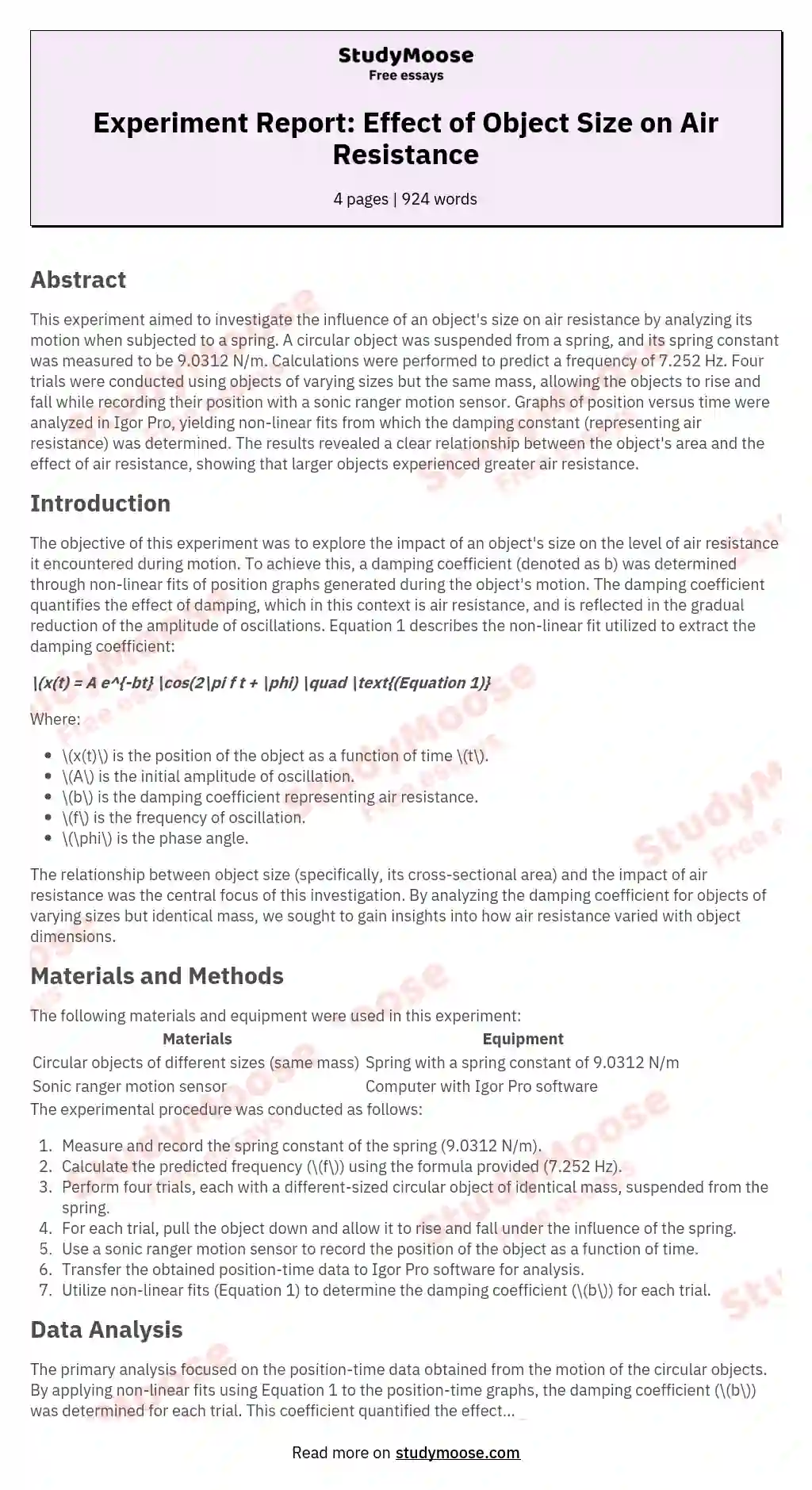# Damped Harmonic Motion Lab Report

Categories: Motion

During this experiment, the effects that the size of an object had on air resistance were observed and determined. To do this, a spring was set up with a circular object hanging at the end. After the spring constant of 9.0312 N/m was measured, equations were used to determine a calculated frequency, that being 7.252 Hz. Four trials—each with a different sized, same massed object—took place where the object was pulled and allowed to rise and fall, while a sonic ranger motion sensor graphed the object’s position.

The graphs created were transferred into Igor Pro, where a non-linear fit was created. From this fit, the damping constant of the object’s motion was given, and the effect of air resistance on the object was determined. A relationship was discovered between the object’s area and the effect air resistance had. The results showed that with a greater area of the object, there was more air resistance on the object.

Get quality help nowWriterBelleVerified writer

Proficient in: Motion4.7 (657)

“ Really polite, and a great writer! Task done as described and better, responded to all my questions promptly too! ”+84 relevant experts are online

Introduction
The goal of this experiment was to observe the effect that the size of an object had on the air resistance shown when the object was in motion. In order to do this, a damping coefficient was determined through non-linear fits of position graphs produced during its motion. The damping coefficient shows the effect that the damping—air resistance—has on the object, shown by a gradual decrease in the size of the amplitude of its oscillations. The damping coefficient is represented by the variable b. Equation 1 shows the non-linear fit used to retrieve the damping coefficient.

Get to Know The Price Estimate For Your Paper
Topic
Number of pages
Email Invalid email